﻿ 基于移动网格的HLL格式求解浅水波方程

# 基于移动网格的HLL格式求解浅水波方程Solving Shallow Water Wave Equation with HLL Scheme Based on Moving Grid

Abstract: In this paper, an HLL scheme based on moving grid is proposed to solve shallow water wave equations. Based on the iterative process, the moving mesh method redistributes the mesh in each iteration by using the equal distribution principle, and then updates its numerical solution by using the conservative interpolation formula, the main idea of this method is to preserve the mass conservation of the numerical solution under each redistribution step. HLL scheme is a numerical flux with good robustness and can eliminate the carbuncle. The time direction is advanced by the third- order strongly stable Runge-Kutta method, and the comparison of numerical results shows that the HLL scheme based on the moving grid has good resolution.

1. 引言

2. 控制方程

${U}_{t}+F{\left(U\right)}_{x}+G{\left(U\right)}_{y}=-S,$ (1)

$U=\left[\begin{array}{c}h\\ hu\\ hv\end{array}\right],F\left(U\right)=\left[\begin{array}{c}hu\\ h{u}^{2}+\frac{1}{2}g{h}^{2}\\ huv\end{array}\right],G\left(U\right)=\left[\begin{array}{c}hv\\ huv\\ h{v}^{2}+\frac{1}{2}g{h}^{2}\end{array}\right],$

$S=\left[\begin{array}{c}0\\ gh\frac{\partial {z}_{b}}{\partial x}+gh{S}_{{f}_{x}}\\ gh\frac{\partial {z}_{b}}{\partial y}+gh{S}_{{f}_{y}}\end{array}\right],$

${z}_{b}$ 表示底部地势函数， $gh\left(\partial {z}_{b}/\partial x\right)$$gh\left(\partial {z}_{b}/\partial y\right)$ 表示水下底部作用力沿x方向和y方向上的分力， $gh{S}_{{f}_{x}}$$gh{S}_{{f}_{y}}$ 为水下底面摩擦力沿x方向和y方向的量， ${S}_{{f}_{x}}$${S}_{{f}_{y}}$ 为x方向和y方向上的摩阻比率，有

${S}_{{f}_{x}}=\frac{u}{{K}^{2}}\frac{{\left({u}^{2}+{v}^{2}\right)}^{1/2}}{{h}^{4/3}},{S}_{{f}_{y}}=\frac{v}{{K}^{2}}\frac{{\left({u}^{2}+{v}^{2}\right)}^{1/2}}{{h}^{4/3}},$

K表示摩擦因数，通常情况取 $K=50$

3. 自适应移动网格法

$\begin{array}{l}{\alpha }_{j+\frac{1}{2},k}\left({\stackrel{\to }{z}}_{j+1,k}^{\left[v\right]}-{\stackrel{\to }{z}}_{j,k}^{\left[v+1\right]}\right)-{\alpha }_{j-\frac{1}{2},k}\left({\stackrel{\to }{z}}_{j,k}^{\left[v+1\right]}-{\stackrel{\to }{z}}_{j-1,k}^{\left[v+1\right]}\right)\\ \text{ }+{\beta }_{j,k+1/2}\left({\stackrel{\to }{z}}_{j,k+1}^{\left[v\right]}-{\stackrel{\to }{z}}_{j-1,k}^{\left[v+1\right]}\right)-{\beta }_{j,k+1/2}\left({\stackrel{\to }{z}}_{j,k}^{\left[v+1\right]}-{\stackrel{\to }{z}}_{j,k-1}^{\left[v+1\right]}\right)=0\end{array}$

${\alpha }_{j±\frac{1}{2},k}=w\left({u}_{j±\frac{1}{2},k}^{\left[v\right]}\right)=w\left(\frac{1}{2}\left({u}_{j±\frac{1}{2},k+\frac{1}{2}}^{\left[v\right]}+{u}_{j±\frac{1}{2},k-\frac{1}{2}}^{\left[v\right]}\right)\right)$

${\beta }_{j,k±\frac{1}{2}}=w\left({u}_{j,k±\frac{1}{2}}^{\left[v\right]}\right)=w\left(\frac{1}{2}\left({u}_{j+\frac{1}{2},k±\frac{1}{2}}^{\left[v\right]}+{u}_{j-\frac{1}{2},k±\frac{1}{2}}^{\left[v\right]}\right)\right)$

2) 新网格上的数值解 $\left\{{u}_{j+1/2,k+1/2}^{\left[v+1\right]}\right\}$ 通过守恒插值进行更新；

3) 重复迭代过程1) 和2) 到指定的迭代步数(3~5步)或直到 $‖{\stackrel{\to }{z}}^{\left[v+1\right]}-{\stackrel{\to }{z}}^{\left[v\right]}‖\le \epsilon$

4. HLL通量格式

${H}^{HLL}=\frac{{S}_{R}^{+}{H}_{k}\left({U}_{L}\right)-{S}_{L}^{-}{H}_{k}\left({U}_{R}\right)}{{S}_{R}^{+}-{S}_{L}^{-}}+\frac{{S}_{R}^{+}{S}_{L}^{-}}{{S}_{R}^{+}-{S}_{L}^{-}}\Delta U,$ (2)

${H}_{k}=\left(F,G\right)\cdot n\left(k=x,y\right),\text{\hspace{0.17em}}\text{\hspace{0.17em}}n=\left({n}_{x},{n}_{y}\right),\text{\hspace{0.17em}}\text{\hspace{0.17em}}\Delta U={U}_{R}-{U}_{L},$

${S}_{R}^{+}=\mathrm{max}\left(0,{S}_{R}\right),\text{\hspace{0.17em}}\text{\hspace{0.17em}}{S}_{L}^{-}=\mathrm{min}\left(0,{S}_{L}\right),$

${S}_{L},{S}_{R}$ 分别表示左波速和右波速，它们的值分别为Jacobi矩阵 ${\stackrel{^}{A}}_{k}=\frac{\partial {H}_{k}}{\partial U}$ 特征值的最小值和最大值，即

${S}_{L}=\mathrm{min}\left({\left({q}_{n}\right)}_{L}-{c}_{L},{\stackrel{^}{q}}_{n}-\stackrel{^}{c}\right),\text{\hspace{0.17em}}\text{\hspace{0.17em}}{S}_{R}=\mathrm{max}\left({\left({q}_{n}\right)}_{R}+{c}_{R},{\stackrel{^}{q}}_{n}+\stackrel{^}{c}\right),$

${\left({q}_{n}\right)}_{L,R}={\left(u,v\right)}_{L,R}\cdot n,\text{\hspace{0.17em}}\text{\hspace{0.17em}}{\stackrel{^}{q}}_{n}=\left(\stackrel{^}{u},\stackrel{^}{v}\right)\cdot n,\text{\hspace{0.17em}}\text{\hspace{0.17em}}{\stackrel{^}{q}}_{t}=\left(\stackrel{^}{u},\stackrel{^}{v}\right)\cdot \left(-{n}_{x},{n}_{y}\right).$

${H}^{\text{HLL}}=\left\{\begin{array}{ll}{F}_{L}\cdot {n}_{x}+{G}_{L}\cdot {n}_{y}\hfill & 0\le {S}_{L},\hfill \\ \frac{{S}_{R}^{+}{H}_{k}\left({U}_{L}\right)-{S}_{L}^{-}{H}_{k}\left({U}_{R}\right)}{{S}_{R}^{+}-{S}_{L}^{-}}+\frac{{S}_{R}^{+}{S}_{L}^{-}}{{S}_{R}^{+}-{S}_{L}^{-}}\Delta U,\hfill & {S}_{L}\le 0\le {S}_{R},\hfill \\ {F}_{R}\cdot {n}_{x}+{G}_{R}\cdot {n}_{y},\hfill & 0\ge {S}_{R}.\hfill \end{array}$ (3)

$\begin{array}{l}{U}^{\left(1\right)}={U}^{n}+\Delta tL\left({U}^{n}\right),\\ {U}^{\left(2\right)}=\frac{3}{4}{U}^{n}+\frac{1}{4}{U}^{\left(1\right)}+\frac{\Delta t}{4}L\left({U}^{\left(1\right)}\right),\\ {U}^{n+1}=\frac{1}{3}{U}^{n}+\frac{2}{3}{U}^{\left(2\right)}+\frac{2}{3}\Delta tL\left({U}^{\left(2\right)}\right).\end{array}$ (4)

5. 数值算例

$\begin{array}{l}h\left(x,y,0\right)=\left\{\begin{array}{l}10,\text{\hspace{0.17em}}\text{\hspace{0.17em}}\text{\hspace{0.17em}}\sqrt{{\left(x-25\right)}^{2}+{\left(y-25\right)}^{2}}<11\\ 1,\text{\hspace{0.17em}}\text{\hspace{0.17em}}\text{\hspace{0.17em}}\text{\hspace{0.17em}}\text{\hspace{0.17em}}\text{others}.\end{array}\\ u\left(x,y,0\right)=v\left(x,y,0\right)=0,\text{\hspace{0.17em}}\text{\hspace{0.17em}}{z}_{b}\left(x,y\right)=0.\end{array}$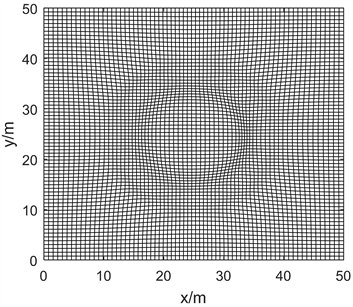Figure 1. Grid evolution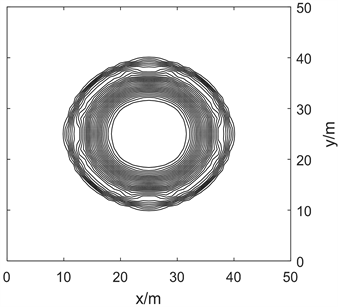(a)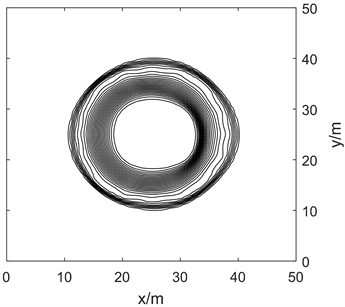(b)

Figure 2. The cylindrical dam-break density contour. (a) The fixed grid diagram; (b) The moving grid diagram

$\begin{array}{l}h\left(x,y,0\right)=\left\{\begin{array}{l}2,\text{\hspace{0.17em}}\text{\hspace{0.17em}}\text{\hspace{0.17em}}\sqrt{{x}^{2}+{y}^{2}}<0.5\\ 1,\text{\hspace{0.17em}}\text{\hspace{0.17em}}\text{\hspace{0.17em}}\text{\hspace{0.17em}}\text{others}.\end{array}\\ u\left(x,y,0\right)=v\left(x,y,0\right)=0,\text{\hspace{0.17em}}\text{\hspace{0.17em}}{z}_{b}\left(x,y\right)=0.\end{array}$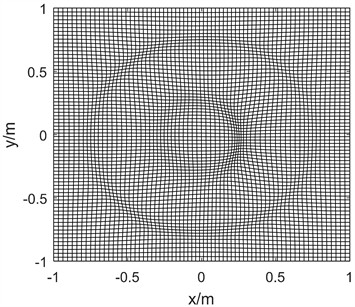Figure 3. Grid evolution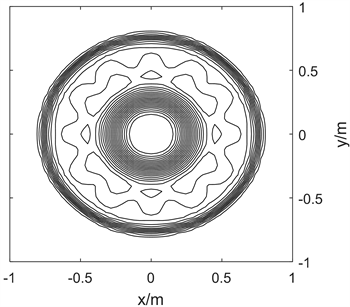(a)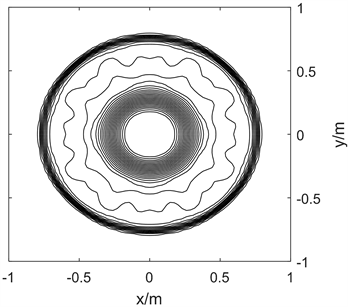(b)

Figure 4. The circular dam-break density contour. (a) The fixed grid diagram; (b) The moving grid diagram

$h\left(x,y,0\right)={z}_{b}\left(x,y,0\right)+\zeta \left(x,y,0\right),$

$u\left(x,y,0\right)=v\left(x,y,0\right)=0$

${z}_{b}\left(x,y\right)=1+0.01\mathrm{cos}\left(\pi x/2\right)\mathrm{cos}\left(\pi y/2\right)$

$CFL=0.45$，时间 $t=0.3$$g=0.98$，控制参数 $\alpha =0.935$，边界条件采用周期性条件。图5表示的是二维潮汐问题密度等值线图，网格演化效果不明显，通过对比可以发现两种格式没有明显差别，均对称性良好，结构清晰。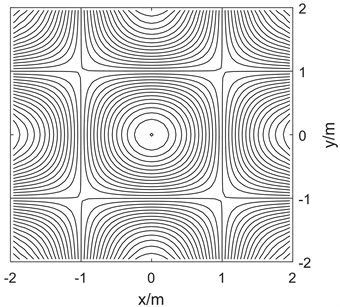(a)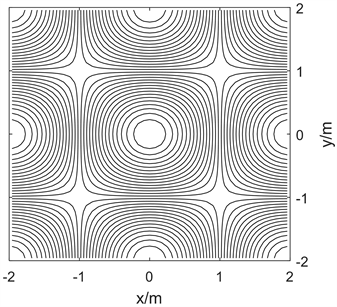(b)

Figure 5. The density contour of tidal. (a) The fixed grid diagram; (b) The moving grid diagram

6. 总结

 Harten, A. and Hyman, J.M. (1983) Self Adjusting Grid Methods for One-Dimensional Hyperbolic Conservation Laws. Journal of Computational Physics, 50, 235-269.
https://doi.org/10.1016/0021-9991(83)90066-9

 Xu, X.H., and Ni, G.X. (2013) A High-Order Moving Mesh Kinetic Scheme Based on WENO Reconstruction for Compressible Flows. Chinese Journal of Computational Physics, 30, 509-514.

 Han, E, Li, J.Q. and Tang, H.Z. (2010) An Adaptive GRP Scheme for Compressible Fluid Flows. Journal of Computational Physics, 229, 1448-1466.
https://doi.org/10.1016/j.jcp.2009.10.038

 Tang, H.Z. and Tang, T. (2003) Adaptive Mesh Methods for One-and Two-Dimensional Hyperbolic Conservation Laws. SIAM Journal on Numerical Analysis, 41, 487-515.
https://doi.org/10.1137/S003614290138437X

 Cheng, X.H., Nie, Y.F. and Cai, L. (2017) Entropy Stable Scheme Based on Moving Grid. Chinese Journal of Computational Physics, 34, 175-182.

 王令, 郑素佩. 基于移动网格的熵稳定格式求解浅水波方程[J]. 水动力学研究与进展: A辑, 2020(2): 188-193.

 郑素佩, 王令, 王苗苗. 求解二维浅水波方程的移动网格旋转通量法[J]. 应用数学和力学, 2020, 41(1): 42-53.
https://doi.org/10.21656/1000-0887.400124

 Nishikawa, H. and Kitamura, K. (2008) Very Simple, Carbuncle-Free, Boundary-Layer-Resolving, Rotated-Hybrid Riemann Solvers. Journal of Computational Physics, 227, 2560-2581.
https://doi.org/10.1016/j.jcp.2007.11.003

 Klingenberg, C., Kurganov, A., Liu, Y.L. and Zenk, M. (2020) Moving-Water Equilibria Preserving HLL-Type Schemes for the Shallow Water Equations. Communications in Mathematical Research, 36, 247-271.
https://doi.org/10.4208/cmr.2020-0013

 贾豆, 郑素佩. 求解二维Euler方程的旋转通量混合格式[J]. 应用数学与力学, 2021, 42(2): 170-179.
https://doi.org/10.21656/1000-0887.410196

 Thanh, M.D., Karim, M.F. and Ismail, A.I.M. (2008) Well-Balanced Scheme for Shallow Water Equations with Arbitrary Topography. International Journal of Dynamical Systems & Different Equations, 1, 196-204.
https://doi.org/10.1504/IJDSDE.2008.019681

 Goetz, C.R., Balsara, D.S. and Dumbser, M. (2018) A Family of HLL-Type Solvers for the Generalized Riemann Problem. Computers & Fluids, 169, 201-212.
https://doi.org/10.1016/j.compfluid.2017.10.028

 Gottlieb, S., Ketcheson, D.I. and Shu, C.W. (2009) High Order Strong Stability Preserving Time Discretization. Journal of Scientific Computing, 38, 251-289.
https://doi.org/10.1007/s10915-008-9239-z

Top